Optics (Reflection Refraction Mirrors)Cheatsheet

Optics Cheat Sheet

Reflection of Light

Reflection is turning back of the light from the surface it hits. Incoming and reflected lights have same angle with the surface. If the surface reflects most of the light then we call such surfaces as mirrors.

Laws of Reflection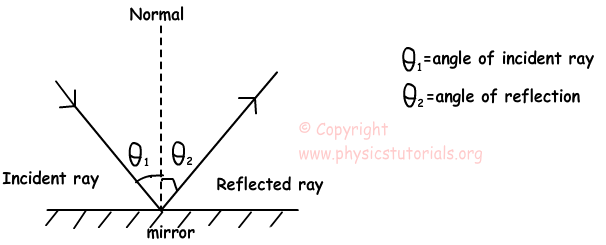First law of reflection states that; Incident ray, reflected ray and Normal to the surface lie in the same plane.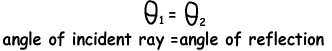Angle of incident ray is equal to the angle of reflection ray.

Plane Mirrors and Image Formation in Plane Mirrors

If the reflecting surface of the mirror is flat then we call this type of mirror as plane mirrors. Light always has regular reflection on plane mirrors.

First look at picture and then follow the steps one by one.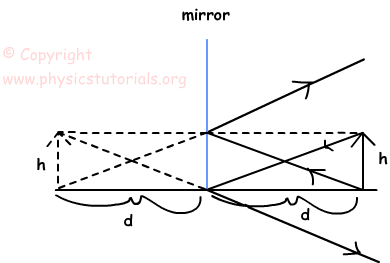In plane mirrors, we use the laws of reflection while drawing the image of the objects. As you see from the picture we send rays from the top and bottom of the object to the mirror and reflect them with the same angle it hits the mirror. The extensions of the reflected rays give us the image of our object. The orientation and height of the image is same as the object. In plane mirrors always virtual image is formed.

Moving Plane Mirror

If the mirror moves with a velocity of V then image of the object moves with the velocity of 2V with the same direction of mirror.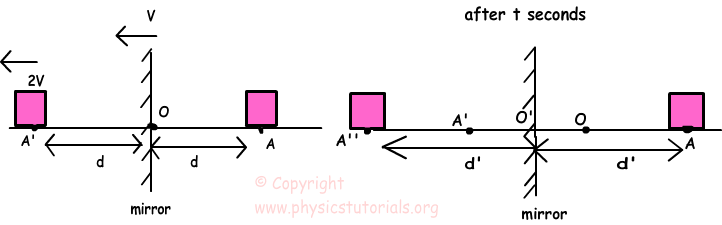If the object moves with a velocity of V then image also moves with same speed but opposite in direction. Pictures given below shows the motion of object and image.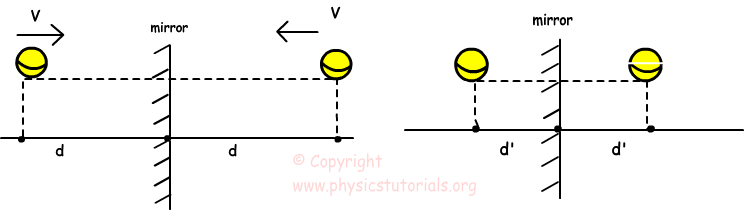Curved Mirrors

We call these types of mirrors also spherical mirrors because they are pieces of a sphere. If the reflecting surface of the mirror is outside of the sphere then we call it convex mirror and if the reflecting surface of it is inside the sphere then we call it concave mirror.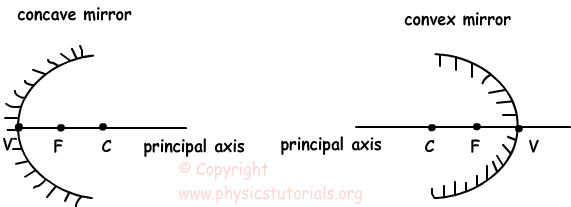Center of Curvature: As you can understand from the name it is the center of the sphere which the mirror is taken from. It is denoted by C in the diagrams.

Principal Axis: Line coming from the center of the sphere to the mirror is called as principal axis.

Vertex: It is the intersection point of the mirror and principal axis. We show it with the letter V in ray diagrams.

Focal Point: For concave mirrors and thin lenses rays coming parallel to the principal axis reflects from the optical device and pass from this point. For convex mirrors and thick lenses rays coming from this point or appear to coming from this point reflect parallel to the principal axis from the optical device. Another explanation for this term is that, it is the point where the image of the object at infinity is formed. It is denoted with the letter F or sometimes f in ray diagrams.

Radius of Curvature: It is the distance between center of the sphere and vertex. We show it with R in ray diagrams.

Mirror Equations of Curved Mirrors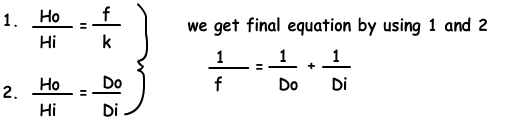Refraction

When light passes from one medium to another medium velocity of it changes and so, its direction changes. We call this change in the direction of light refraction.

Refractive Index

It is the ratio of the speed of light in vacuum to the speed of the light in given medium. Refractive index of medium A is given below;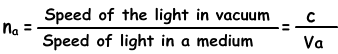The Laws of Refraction

• Incident ray, reflected ray, refracted ray and the normal of the system lie in the same plane.
• Incident ray, coming from one medium to the boundary of another medium, is refracted with a rule derived from a physicist Willebrord Snellius. He found that there is a constant relation between the angle of incident ray and angle of refracted ray. This constant is the refractive index of second medium relative to the first medium. He gives the final form of this equation like;
•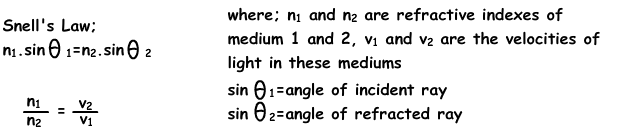Critical Angle and Total ReflectionAccording to the angle of incident ray, at one point it does not refract but goes parallel to the boundary of the mediums. We called this angle as critical angle. If the angle of incident ray is larger than the critical angle then it does not refract but it does total reflection.

Apparent Depth Real Depth

We see the objects under water closer than their real depth to the surface.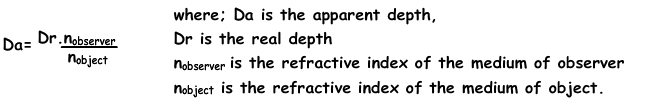Optics Exams and Solutions

Author: